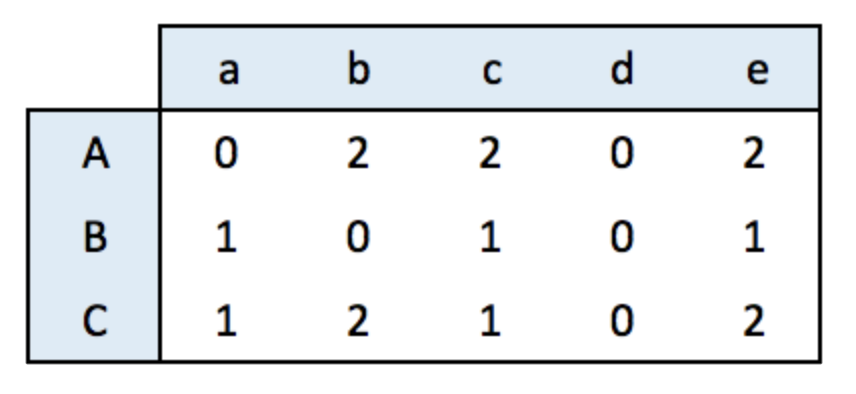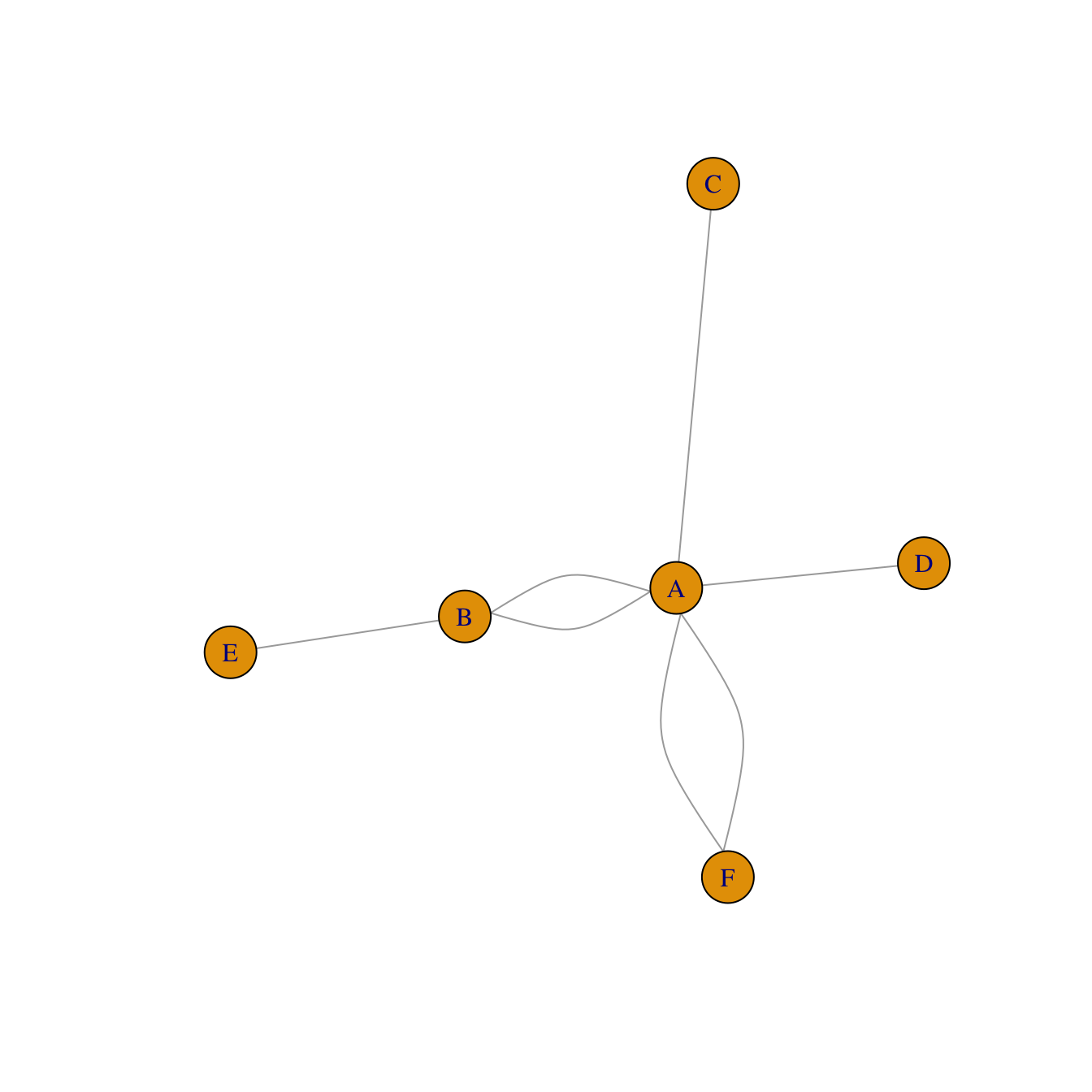# Network chart with R and igraph from any type of input

This post explains how to get started with the `igraph` package from any type of input. It shows how to build a network diagram from adjacency matrix, edge list, litteral list and more.

An adjacency matrix is a square matrix where individuals in rows and columns are the same.

It’s typically the kind of matrix you get when calculating the correlation between each pair of individual. In this example, we have 1 connection from E to C, and 2 connections from C to E. By default, we get an unweighted and oriented network.``````#library
library(igraph)

# Create data
set.seed(10)
data <- matrix(sample(0:2, 25, replace=TRUE), nrow=5)
colnames(data) = rownames(data) = LETTERS[1:5]

# build the graph object

# plot it
plot(network)``````

# Incidence matrix

An incidence matrix is not square and entities provided in rows and columns are not necessary the same.

Note: by default, the graph object is directed from rows to columns.``````# lib
library(igraph)

# data
set.seed(1)
data <- matrix(sample(0:2, 15, replace=TRUE), nrow=3)
colnames(data) <- letters[1:5]
rownames(data) <- LETTERS[1:3]

# create the network object
network <- graph_from_incidence_matrix(data)

# plot it
plot(network)``````

# Edge list

An edge list has 2 columns. Each row represents a connection between an origin (first column) and a destination (left column).

Note: this kind of input often goes with another dataframe providing features for each node. (See this post). It is also possible to add information concerning each link in the edge list.``````# create data:
source=c("A","A", "A", "A", "A","F", "B"),
target=c("B","B", "C", "D", "F","A","E")
)

# create the network object

# plot it
plot(network)``````

# Literal List of connections

Last option that is less often used: a litteral list of the connection. Can be understand by `igraph` thanks to the `graph_from_literal()` function.``````# create data:
network <- graph_from_literal( A-B-C-D, E-A-E-A, D-C-A, D-A-D-C )

# plot it
plot(network)``````

Related chart types

## Contact

This document is a work by Yan Holtz. Any feedback is highly encouraged. You can fill an issue on Github, drop me a message on Twitter, or send an email pasting yan.holtz.data with gmail.com.